asm.js：面向未来的开发

18 篇文章 0 订阅

What’s asm.js

On this page, we match two chess engines against one another. They are identical in nearly every detail, including source code! The only difference is that one has a flag set to be interpreted as asm.js, a specialized and strict JavaScript subset that allows JavaScript engines to employ specialized compilation to dramatically speed up execution.
Each turn is limited to 200ms – because the asm.js-optimized engine has a significant performance advantage, it can evaluate more moves per turn and has a substantially higher likelihood of victory. You can adjust the turn length and other variables in the demo to see how they affect the outcome of the game.主角登场

asm.js不是一门新的语言，而是JavaScript的一个子集。由Mozilla于2013年提出，主要为了提升JS引擎执行代码的速度。通俗来说，同样是js代码，符合asm.js规范的代码对JS引擎更加友好，JS引擎在解释执行这些代码更加省心（例如不用进行变量类型推断了），也就更加快。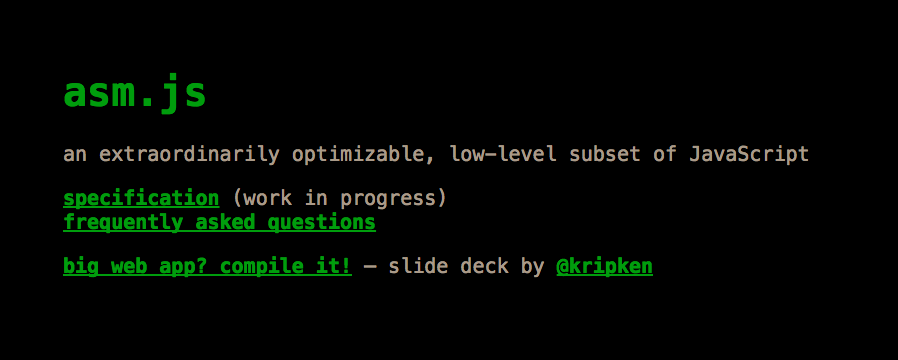an extraordinarily optimizable, low-level subset of JavaScript

asm.js、js、C++

C++

// For x = 1 to infinity:
// if x not divisible by any member of an initially empty list of primes,
// add x to the list until we have 25,000
//
// x从1递增到无穷，如果x不能整除list里的所有数，
// 那么将x添加到list，直到list中有25,000个数
#include <stdio.h>

class Primes {
public:
int getPrimeCount() const { return prime_count; }
int getPrime(int i) const { return primes[i]; }
void addPrime(int i) { primes[prime_count++] = i; }

bool isDivisibe(int i, int by) { return (i % by) == 0; }

bool isPrimeDivisible(int candidate) {
for (int i = 1; i < prime_count; ++i) {
if (isDivisibe(candidate, primes[i])) return true;
}
return false;
}

private:
volatile int prime_count;
volatile int primes;
};

int main() {
Primes p;
int c = 1;
while (p.getPrimeCount() < 25000) {
if (!p.isPrimeDivisible(c)) {
}
c++;
}
printf("%d\n", p.getPrime(p.getPrimeCount()-1));
}

js

// For x = 1 to infinity:
// if x not divisible by any member of an initially empty list of primes,
// add x to the list until we have 25,000
//
// x从1递增到无穷，如果x不能整除list里的所有数，
// 那么将x添加到list，直到list中有25,000个数

// 开始打点
// console.log("start");
var startTime = new Date();

function Primes() {
this.prime_count = 0;
this.primes = new Array(25000);
this.getPrimeCount = function() { return this.prime_count; }
this.getPrime = function(i) { return this.primes[i]; }
this.primes[this.prime_count++] = i;
}

this.isPrimeDivisible = function(candidate) {
for (var i = 1; i <= this.prime_count; ++i) {
if ((candidate % this.primes[i]) == 0) return true;
}
return false;
}
};

function main() {
p = new Primes();
var c = 1;
while (p.getPrimeCount() < 25000) {
if (!p.isPrimeDivisible(c)) {
}
c++;
}
console.log(p.getPrime(p.getPrimeCount()-1));
}

main();

// 结束打点
// console.log("end");
console.log("raw_js.js: ",(new Date() - startTime)/1000,"s");

asm.js规范（对，你没看错，下面就是js代码）

function _main() {
var $0 = 0,$1 = 0, $10 = 0,$11 = 0, $2 = 0,$3 = 0, $4 = 0,$5 = 0, $6 = 0,$7 = 0, $8 = 0,$9 = 0, $c = 0,$p = 0, $vararg_buffer = 0, label = 0, sp = 0; sp = STACKTOP; STACKTOP = STACKTOP + 100016|0; if ((STACKTOP|0) >= (STACK_MAX|0)) abort();$vararg_buffer = sp;
$p = sp + 8|0;$0 = 0;
$c = 1; while(1) {$1 = (__ZNK6Primes13getPrimeCountEv($p)|0);$2 = ($1|0)<(25000); if (!($2)) {
break;
}
$3 =$c;
$4 = (__ZN6Primes16isPrimeDivisibleEi($p,$3)|0); if (!($4)) {
$5 =$c;
__ZN6Primes8addPrimeEi($p,$5);
}
$6 =$c;
$7 = (($6) + 1)|0;
$c =$7;
}
$8 = (__ZNK6Primes13getPrimeCountEv($p)|0);
$9 = (($8) - 1)|0;
$10 = (__ZNK6Primes8getPrimeEi($p,$9)|0); HEAP32[$vararg_buffer>>2] = $10; // 结束打点 // console.log("end"); console.log("asm.js: ",(new Date() - startTime)/1000, "s"); (_printf(672,$vararg_buffer)|0);
$11 =$0;
STACKTOP = sp;return ($11|0); } function __ZNK6Primes13getPrimeCountEv($this) {
$this =$this|0;
var $0 = 0,$1 = 0, $2 = 0, label = 0, sp = 0; sp = STACKTOP; STACKTOP = STACKTOP + 16|0; if ((STACKTOP|0) >= (STACK_MAX|0)) abort();$0 = $this;$1 = $0;$2 = HEAP32[$1>>2]|0; STACKTOP = sp;return ($2|0);
}
function __ZN6Primes16isPrimeDivisibleEi($this,$candidate) {
$this =$this|0;
$candidate =$candidate|0;
var $$expand_i1_val = 0,$$expand_i1_val2 = 0, $$pre_trunc = 0, 0 = 0, 1 = 0, 10 = 0, 11 = 0, 12 = 0, 13 = 0, 14 = 0, 15 = 0, 2 = 0, 3 = 0, 4 = 0, 5 = 0, 6 = 0, 7 = 0, 8 = 0, 9 = 0, i = 0; var label = 0, sp = 0; sp = STACKTOP; STACKTOP = STACKTOP + 16|0; if ((STACKTOP|0) >= (STACK_MAX|0)) abort(); 0 = sp + 12|0; 1 = this; 2 = candidate; 3 = 1; i = 1; while(1) { 4 = i; 5 = HEAP32[3>>2]|0; 6 = (4|0)<(5|0); if (!(6)) { label = 6; break; } 7 = 2; 8 = i; 9 = (((3)) + 4|0); 10 = ((9) + (8<<2)|0); 11 = HEAP32[10>>2]|0; 12 = (__ZN6Primes10isDivisibeEii(3,7,11)|0); if (12) { label = 4; break; } 13 = i; 14 = ((13) + 1)|0; i = 14; } if ((label|0) == 4) {$$expand_i1_val = 1;
HEAP8[$0>>0] = $$expand_i1_val;$$pre_trunc = HEAP8[$0>>0]|0;
$15 = $$pre_trunc&1; STACKTOP = sp;return (15|0); } else if ((label|0) == 6) {$$expand_i1_val2 = 0; HEAP8[$0>>0] = $$expand_i1_val2;$$pre_trunc = HEAP8[$0>>0]|0;$15 = pre_trunc&1;
STACKTOP = sp;return ($15|0); } return (0)|0; } function __ZN6Primes8addPrimeEi($this,$i) {$this = $this|0;$i = $i|0; var$0 = 0, $1 = 0,$2 = 0, $3 = 0,$4 = 0, $5 = 0,$6 = 0, $7 = 0, label = 0, sp = 0; sp = STACKTOP; STACKTOP = STACKTOP + 16|0; if ((STACKTOP|0) >= (STACK_MAX|0)) abort();$0 = $this;$1 = $i;$2 = $0;$3 = $1;$4 = HEAP32[$2>>2]|0;$5 = (($4) + 1)|0; HEAP32[$2>>2] = $5;$6 = ((($2)) + 4|0);$7 = (($6) + ($4<<2)|0);
HEAP32[$7>>2] =$3;
STACKTOP = sp;return;
}
function __ZNK6Primes8getPrimeEi($this,$i) {
$this =$this|0;
$i =$i|0;
var $0 = 0,$1 = 0, $2 = 0,$3 = 0, $4 = 0,$5 = 0, $6 = 0, label = 0, sp = 0; sp = STACKTOP; STACKTOP = STACKTOP + 16|0; if ((STACKTOP|0) >= (STACK_MAX|0)) abort();$0 = $this;$1 = $i;$2 = $0;$3 = $1;$4 = ((($2)) + 4|0);$5 = (($4) + ($3<<2)|0);
$6 = HEAP32[$5>>2]|0;
STACKTOP = sp;return ($6|0); } function __ZN6Primes10isDivisibeEii($this,$i,$by) {
$this =$this|0;
$i =$i|0;
$by =$by|0;
var $0 = 0,$1 = 0, $2 = 0,$3 = 0, $4 = 0,$5 = 0, $6 = 0, label = 0, sp = 0; sp = STACKTOP; STACKTOP = STACKTOP + 16|0; if ((STACKTOP|0) >= (STACK_MAX|0)) abort();$0 = $this;$1 = $i;$2 = $by;$3 = $1;$4 = $2;$5 = (($3|0) % ($4|0))&-1;
$6 = ($5|0)==(0);
STACKTOP = sp;return (\$6|0);
}

生成asm.js代码

Emscripten的作者之一kripken也是asm.js规范的草案起稿者之一。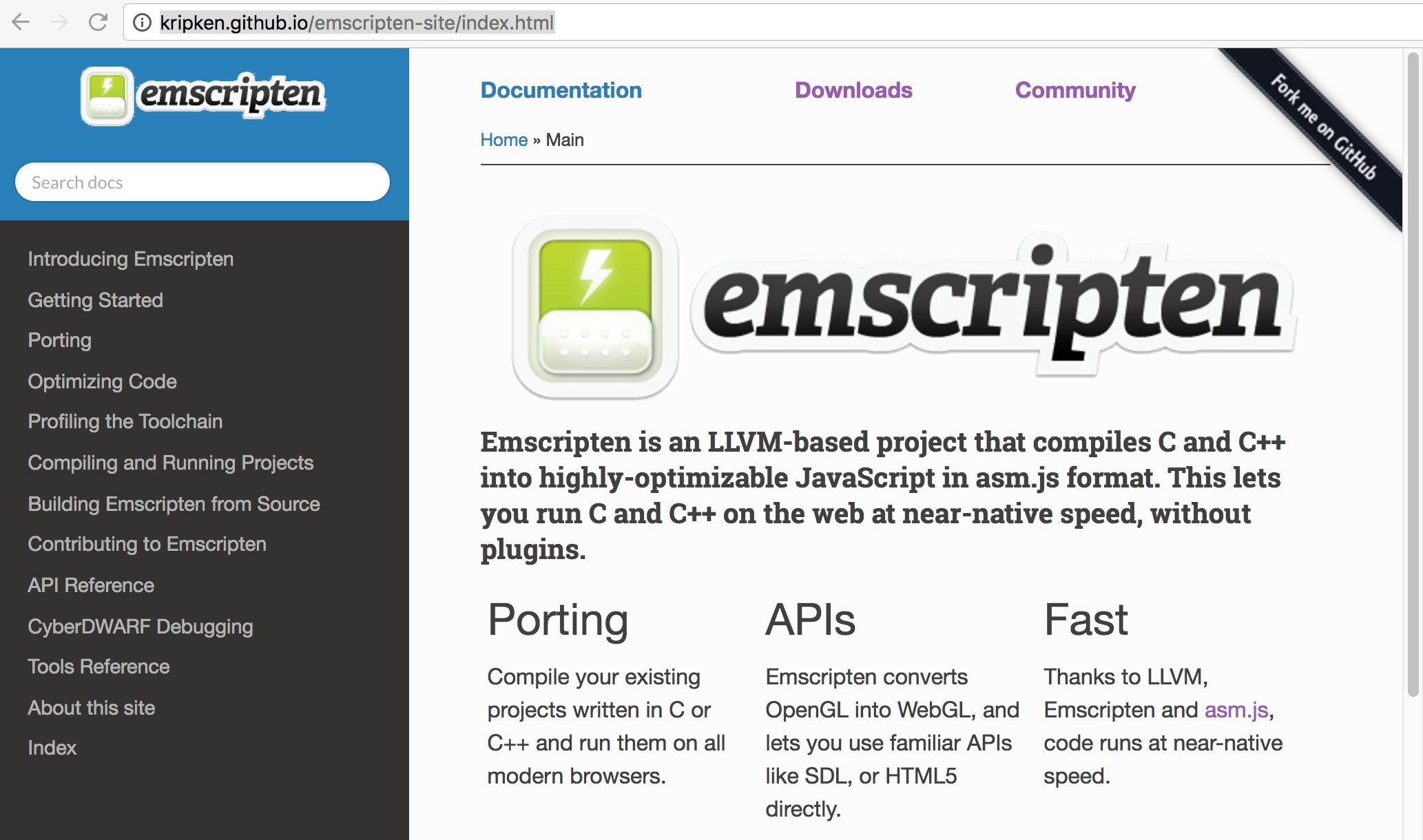Emscripten是一个基于LLVM（Low Level Virtual Machine，编译器框架系统）的项目，它能将C和C++编译成浏览器高度可优化的asm.js代码。这使得我们能够在web环境中以接近原生的速度运行C和C++，且不需要额外的浏览器插件。

性能

.././emcc raw_c.cpp -o asm.js
.././emcc -O1 raw_c.cpp -o asm_O1.js
.././emcc -O2 raw_c.cpp -o asm_O2.js
.././emcc -O3 raw_c.cpp -o asm_O3.js
.././emcc -Oz raw_c.cpp -o asm_Oz.js

对比

① node环境：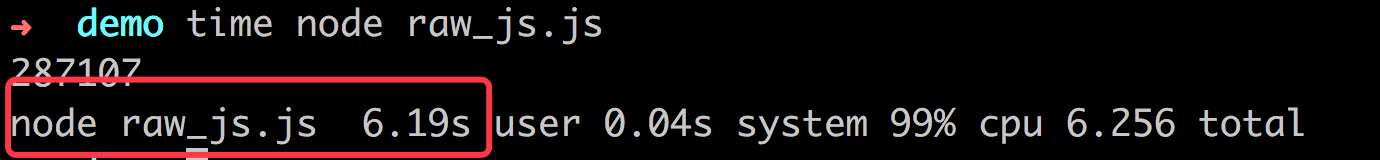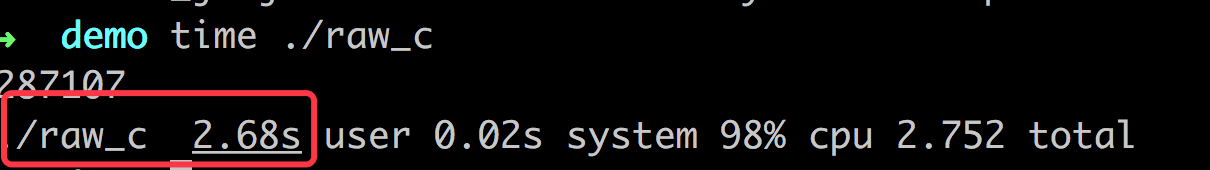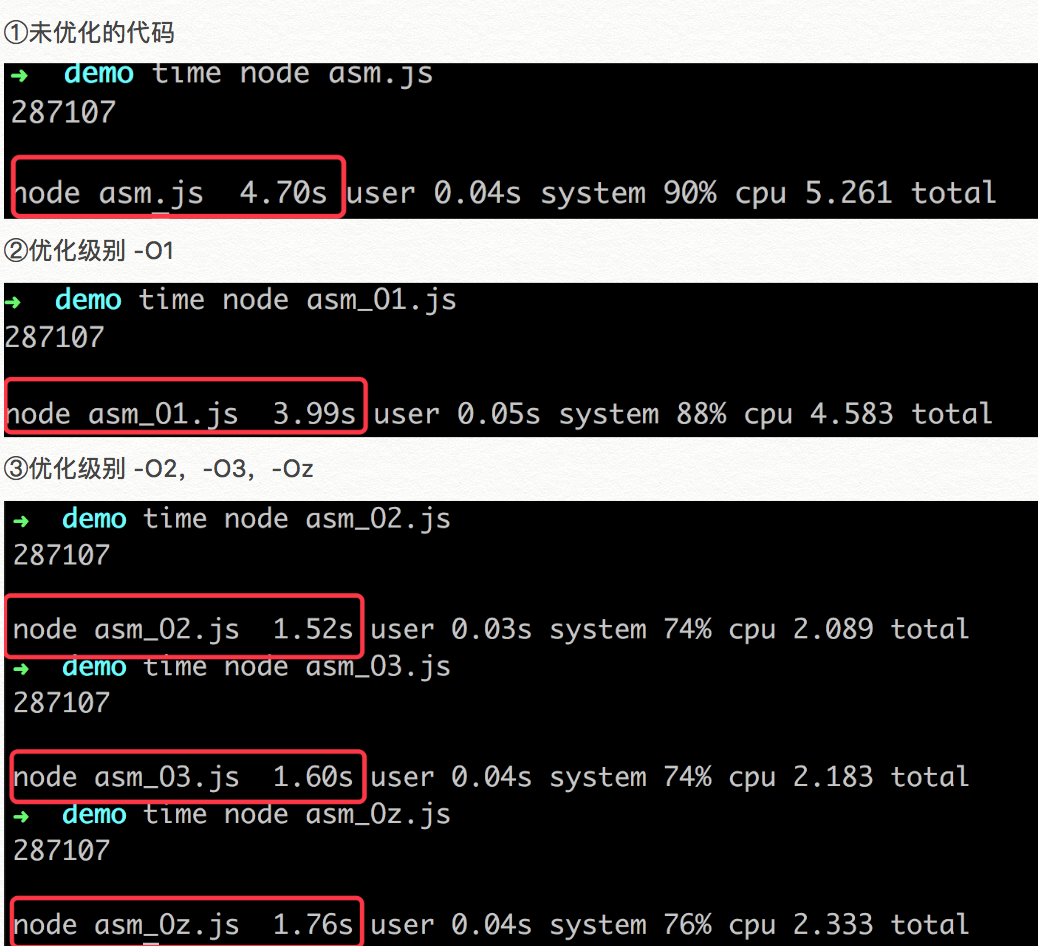② 浏览器环境（Chrome vs Safari）：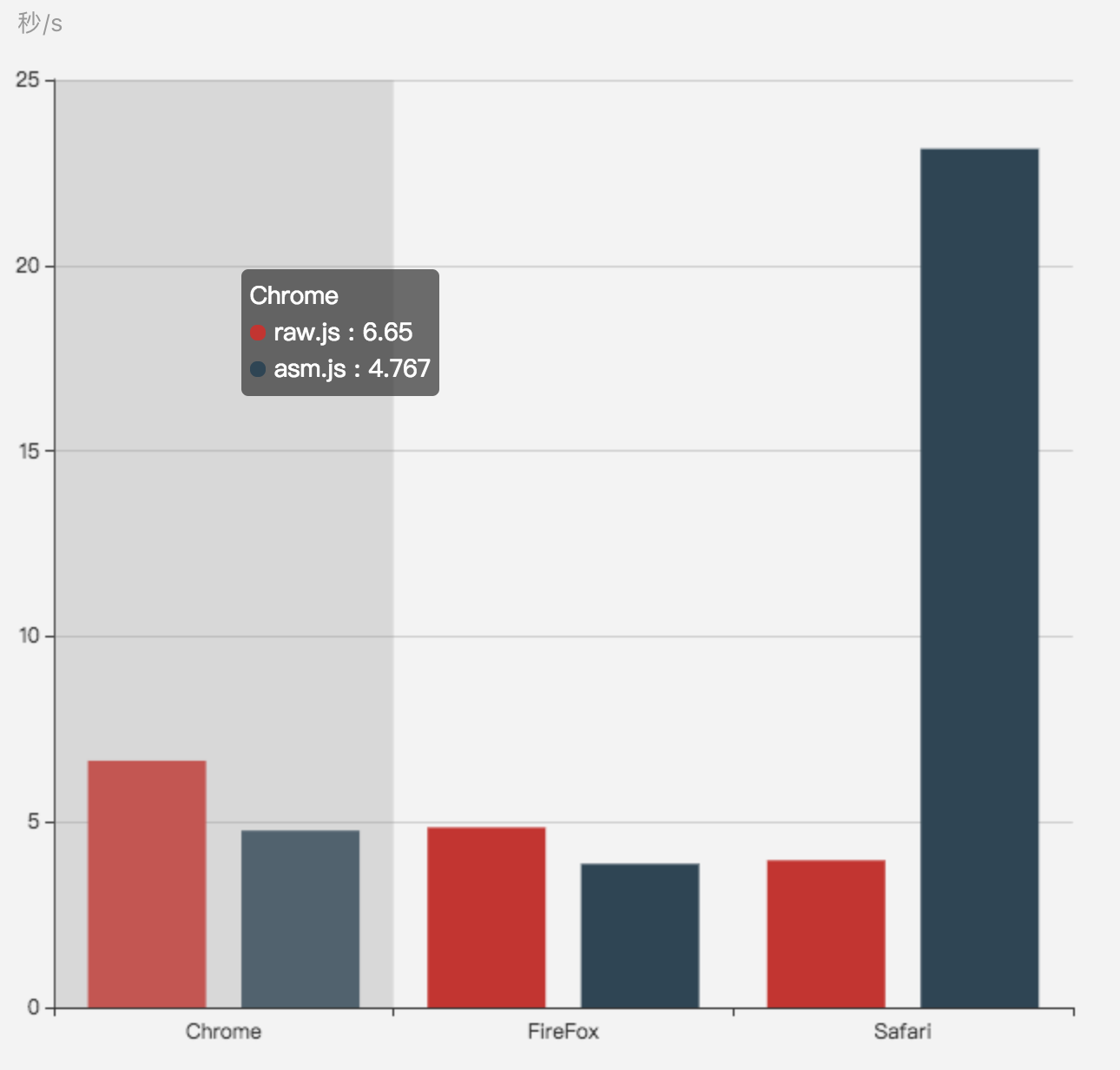为什么快

“来来来，兄弟们，今天我们来商量一件事，是这样的，昨晚我的Windows电脑坏了，手上的Mac又打不了《英雄联盟》 ，我想要是能在Web里打《英雄联盟》那多好，这样就不管什么Windows呀，Mac呀，Ubuntu…打开浏览器马上开撸，爽不爽？然后我昨晚就试着在web上写起了游戏来，结果一运行，我的天，这帧率怎么补刀，超神也别想了。于是苦闷得我整晚睡不着开始思考人生，首要问题还是，js在web上怎么运行这么慢呀？

“我一想，王侯将相宁有种乎，凭啥C++写起游戏来这么快，我大JavaScript就玩不溜？于是ASM.js规范诞生了。兄弟们呀，你们按照这个规范做，保准跟着大哥在浏览器里面打撸把把超神呀！”

规范or理论

asm.js规范入手，快主要得益于以下几方面：

• 数据类型确定 (使用位运算等限制数据类型)
• “use asm”指示浏览器跳过数据类型等验证
• 合法的asm.js代码能进行AOT提前编译处理（其中能实现复杂的性能优化）
• 没有垃圾回收机制，内存的分配和回收时机都是手动确定的

AOT编译：在运行前预编译（对比JIT：在运行中编译）

实现上

1，FireFox与Microsoft Edge

2，Chrome

3，Safari

There is no “use asm” mode in JavaScriptCore. Instead WebKit integrates ASM.js optimizations directly in the optimizer. As a result, it is possible to mix ASM-style typing with regular code and still get great performance and power efficiency.

JavaScriptCore(Safari使用的JavaScript引擎)中不支持“use asm”模式。然而，Webkit直接将asm.js的优化集成到优化器中，将来，有可能将asm.js代码与常规代码结合起来，以达到更好的性能同时更加节约能耗。

工程相关

兼容性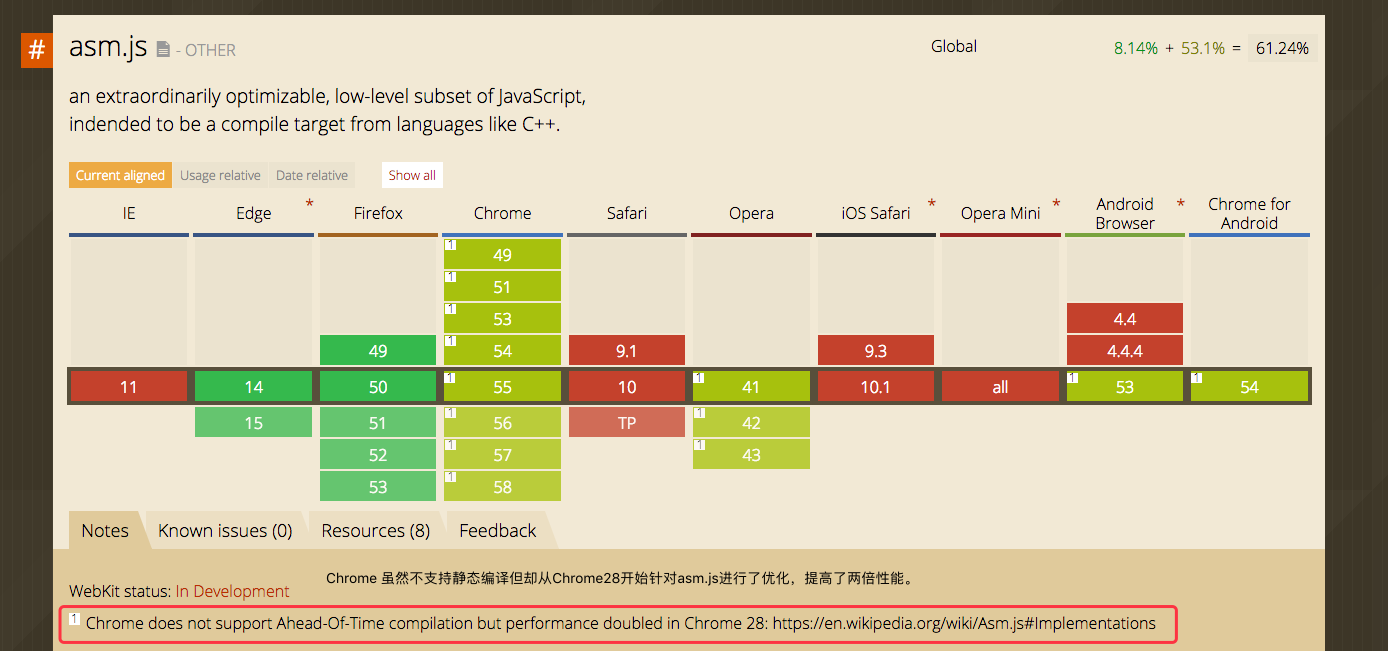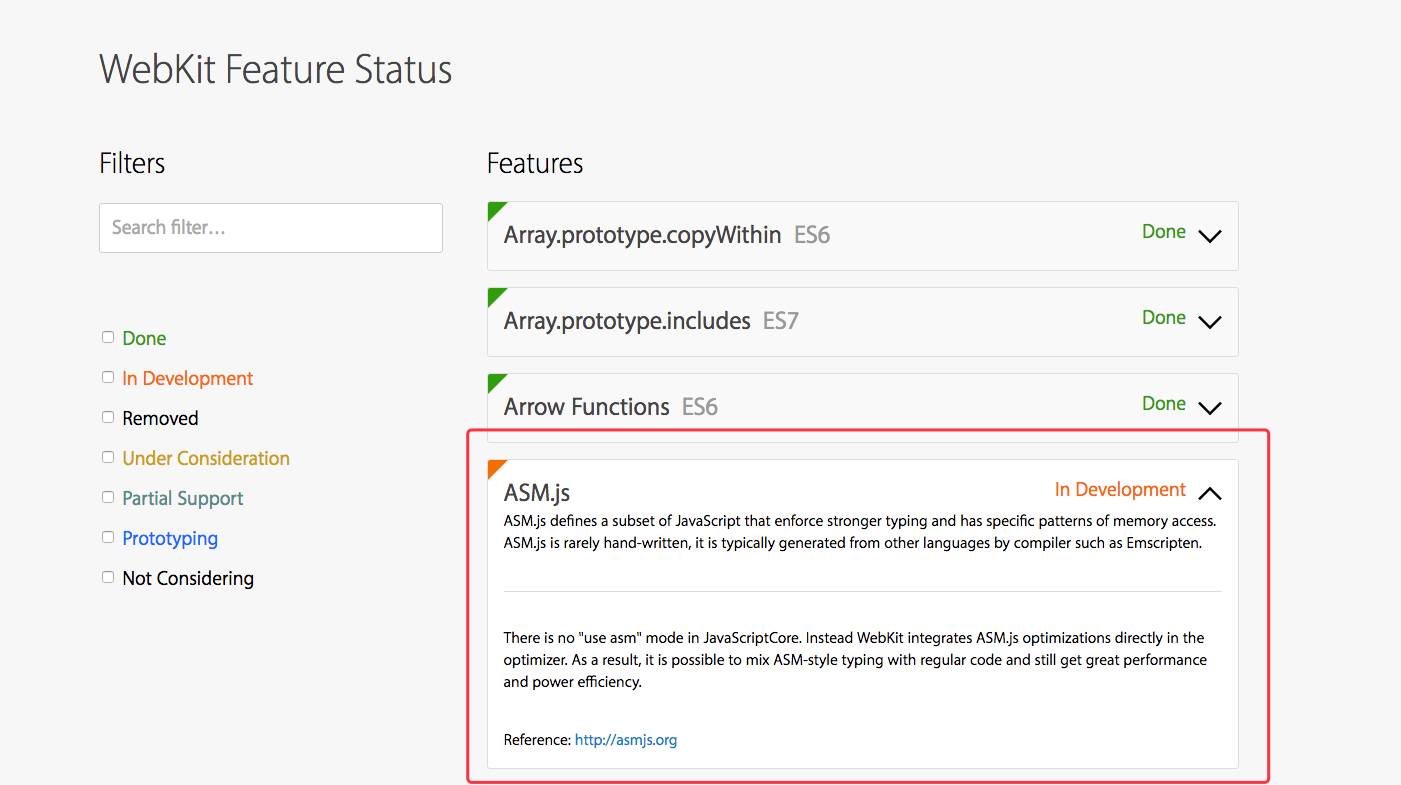打包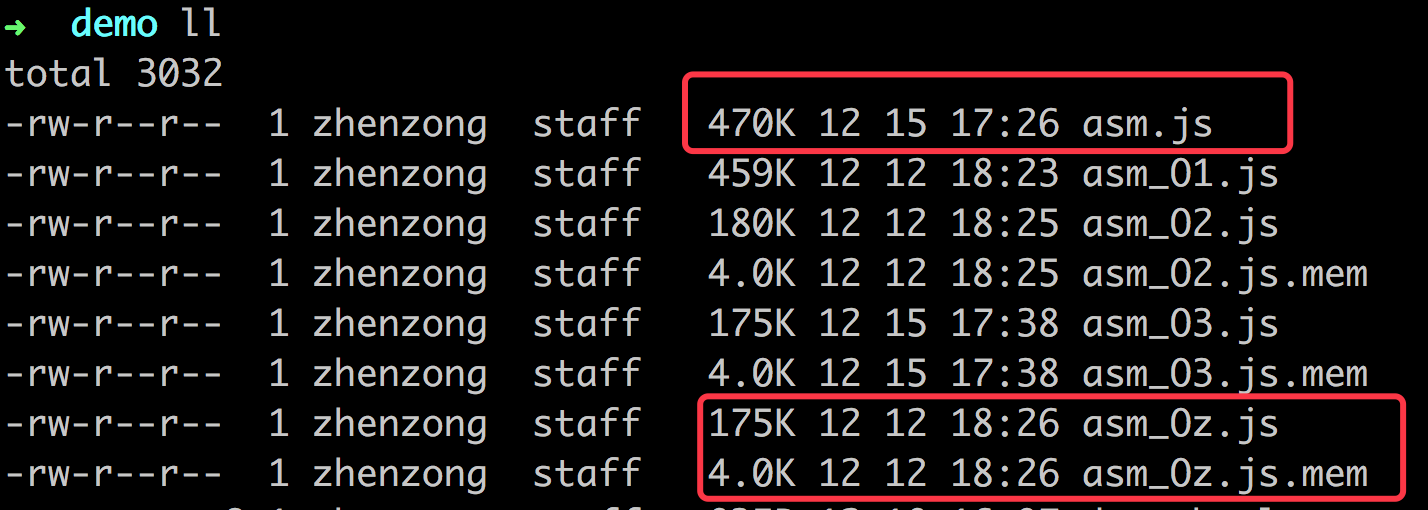C++与JS交互

C++与JavaScript的交互

想象

JavaScript之所以深得大家喜爱，是因为它能运行在所有浏览器上，而web形态是目前连接性，传递性最强的形态。而JavaScript能做什么也一定程度上决定了我们在web上能做什么。那么如果现在我们利用Emscripten能将其他语言编译成可高性能执行的JavaScript，会发生什么？例如，将C++编译器编译成asm运行在浏览器上，那么任何的C++程序是不是就能实时地在浏览器中编译运行？

else

JavaScript的诞生和死亡

12-26144909-111099
09-241841
09-30414
06-29124
03-042134
04-239260
05-212441
07-05240
06-1183
07-12
12-311405Sheldon_Law

¥2 ¥4 ¥6 ¥10 ¥20余额支付 (余额：-- )扫码支付获取中扫码支付点击重新获取扫码支付1.余额是钱包充值的虚拟货币，按照1:1的比例进行支付金额的抵扣。
2.余额无法直接购买下载，可以购买VIP、C币套餐、付费专栏及课程。余额充值